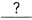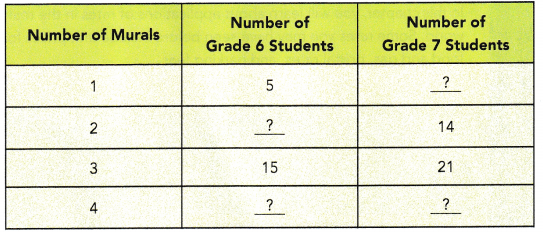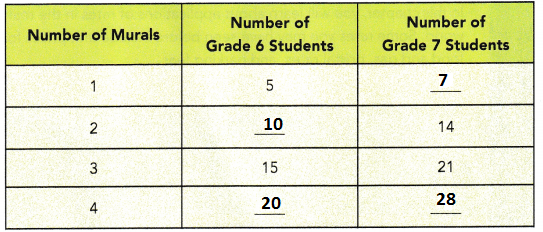# Math in Focus Grade 6 Chapter 4 Review Test Answer Key

Go through the Math in Focus Grade 6 Workbook Answer Key Chapter 4 Review Test to finish your assignments.

## Math in Focus Grade 6 Course 1 A Chapter 4 Review Test Answer Key

Concepts and Skills

Write each ratio in simplest form.

Question 1.
8 : 24
1 : 3
Explanation:
The simplest form of 8 : 24 is 1 : 3
Divide each side by the highest common factor.
That is the highest number which divides evenly into both numbers.
G.C.M for 8 and 24 is 8

Question 2.
6 : 20
3 : 10
Explanation:
The simplest form of 6 : 20 is 3 : 10
Divide each side by the highest common factor.
That is the highest number which divides evenly into both numbers.
G.C.M for 6 and 20 is 2

Question 3.
14 : 49
2 : 7
Explanation:
The simplest form of 14 : 49 is 2 : 7
Divide each side by the highest common factor.
That is the highest number which divides evenly into both numbers.
G.C.M for 14 and 49 is 7

Question 4.
27 : 72
3 : 8
Explanation:
The simplest form of 27 : 72 is 3 : 8
Divide each side by the highest common factor.
That is the highest number which divides evenly into both numbers.
G.C.M for 27 and 72 is 9

Question 5.
14 : 49
2 : 7
Explanation:
The simplest form of 14 : 49 is 2 : 7
Divide each side by the highest common factor.
That is the highest number which divides evenly into both numbers.
G.C.M for 14 and 49 is 7

Question 6.
45 : 30
3 : 2
Explanation:
The simplest form of 45 : 30 is 3 : 2
Divide each side by the highest common factor.
That is the highest number which divides evenly into both numbers.
G.C.M for 45 and 30 is 15

Question 7.
27 : 72
3 : 8
Explanation:
The simplest form of 27 : 72 is 3 : 8
Divide each side by the highest common factor.
That is the highest number which divides evenly into both numbers.
G.C.M for 27 and 72 is 9

Question 8.
64 : 56
8 : 7
Explanation:
The simplest form of 64 : 56 is 8 : 7
Divide each side by the highest common factor.
That is the highest number which divides evenly into both numbers.
G.C.M for 64 and 56 is 8

Find the missing term in each pair of equivalent ratios.

Question 9.
1 : 3 = 6 :Explanation:
product of extremes = product of means
a:b = c:d
$$\frac{a}{b}$$ = $$\frac{c}{d}$$
a = 1, b = 3, c = 6, d = x
1 x x = 3 x 6
x = 18

Question 10.
4 : 7 =: 21
Explanation:
product of extremes = product of means
a:b = c:d
$$\frac{a}{b}$$ = $$\frac{c}{d}$$
a = 4, b = 7, c = x, d = 21
4 x 21 = 7 x x
84 = 7x
x = $$\frac{84}{7}$$
x = 12

Question 11.
25 : 15 =: 3
Explanation:
product of extremes = product of means
a:b = c:d
$$\frac{a}{b}$$ = $$\frac{c}{d}$$
a = 25, b = 15, c = x, d = 3
25 x 3 = 15 x x
15x = 75
x = $$\frac{75}{15}$$
x = 5
Question 12.
54 : 36 = 18 :Explanation:
product of extremes = product of means
a:b = c:d
$$\frac{a}{b}$$ = $$\frac{c}{d}$$
a = 54, b = 36, c = 18, d = x
54 x x = 36 x 18
54x = 648
x = $$\frac{648}{54}$$
x = 12

Question 13.
4 := 20 : 25
Explanation:
product of extremes = product of means
a:b = c:d
$$\frac{a}{b}$$ = $$\frac{c}{d}$$
a = 4, b = x, c = 20, d = 25
4 x 25 = x x 20
100 = 20x
x = $$\frac{100}{20}$$
x = 5

Question 14.: 9 = 48 : 72
Explanation:
product of extremes = product of means
a:b = c:d
$$\frac{a}{b}$$ = $$\frac{c}{d}$$
a = x, b = 9, c = 48, d = 72
x x 72 = 9 x 48
72x = 432
x = $$\frac{432}{72}$$
x = 6

Question 15.
28 := 4 : 6
Explanation:
product of extremes = product of means
a:b = c:d
$$\frac{a}{b}$$ = $$\frac{c}{d}$$
a = 28, b = x, c = 4, d = 6
28 x 6 = x x 4
168 = 4x
x = $$\frac{168}{4}$$
x = 42

Question 16.: 36 = 21 : 12
Explanation:
product of extremes = product of means
a:b = c:d
$$\frac{a}{b}$$ = $$\frac{c}{d}$$
a = x, b = 36, c = 21, d = 12
x x 12 = 36 x 21
12x = 756
x = $$\frac{756}{12}$$
x = 63

Problem Solving

Question 17.
The city has 28 fire engines and 36 fire trucks. Find the ratio of the number of fire engines to the number of fire trucks in simplest form.
7 : 9
Explanation:
The city has 28 fire engines and 36 fire trucks.
Ratio is 28 : 36
The ratio of the number of fire engines to the number of fire trucks in simplest form.
7 : 9

Question 18.
Of the 80 students who signed up for after school clubs, 16 students signed up for the art club, and the rest signed up for other clubs. Find the ratio of the number of students who signed up for the art club to the number of students who signed up for other dubs. Give your answer in simplest form.
1 : 4
Explanation:
80 students who signed up for after school clubs,
16 students signed up for the art club,
the rest signed up for other clubs = 80 – 16 = 64
The ratio of the number of students who signed up for the art club to the number of students who signed up for other dubs are 16 : 64
simplest form of ratio is 1 : 4

Question 19.
On Saturday, Alison used her cell phone for 36 minutes. On Sunday, she used her cell phone for 18 minutes more than on Saturday. Find the ratio of the number of minutes Alison used on Saturday to the total number of minutes on Saturday and Sunday. Give your answer in simplest form.
2 : 5
Explanation:
On Saturday 36 min
On Sunday 36 + 18 = 54 min
the number of minutes Alison used on Saturday to the total number of minutes on Saturday and Sunday is
36 + 54 =90 min
the ratio is 36 : 90 = 2 : 5

Question 20.
Daniel is 12 years old. Elliot is 15 years older than Daniel. Frank is 3 years younger than Elliot. Find the ratio of Daniel’s age to Frank’s age. Give your answer in simplest form.
1: 2
Explanation:
Daniel’s age is 12 years
Elliots age is 15 + 12 = 27 years
Franks age is 27 -3 = 24 years
the ratio of Daniel’s age to Frank’s age
12 : 24
1 : 2

Question 21.
The ratio of the number of left-handed batters to the number of right-handed batters is 5 : 8. There are 45 left-handed batters.
a) How many right-handed batters are there?
Explanation:
45 : 5  = x : 8
x =(45 x 8) /5 = 72

b) Find the ratio of the number of left-handed batters to the total number of batters. Give your answer in simplest form.
5 : 13
Explanation:
45 + 72 = 117 total number of batters
45 : 117
5 : 13

Question 22.
Mrs. Johnson gave a sum of money to her son and daughter in the ratio 5 : 6. Her daughter received $2,400. How much did Mrs. Johnson give away in all? Answer:$2000
Explanation:
son and daughter in the ratio 5 : 6
6 : x = 5 x 2400
x = (5x 2400)/6 = 2000

Question 23.
The ratio of the number of boys to the number of girls in a school is 5 : 7. If there are 600 students in the school, how many girls are there?
350 girls
Explanation:
ratio of boys and girls = 5 + 7 = 12
total students = 600
number of girls =7 x 600/12 = 50 x 7 = 350

Question 24.
The murals in a school are painted by its grade 6 and grade 7 students. The number of mural painters from grade 6 and the number of mural painters from grade 7 is the same for each mural in the school. Copy and complete the table.Explanation:
The number of mural painters from grade 6 and the number of mural painters from grade 7 is the same for each mural in the school
Each time the number of Murals in Garde 6 increased with 5 multiple.
At the same time in Grade 7 also increased with 7 multiple.

Question 25.
A sum of money was shared among Aaron, Ben, and Charles in the ratio 2 : 5 : 7. If Charles’s share was $1,180 more than Aaron’s share, what was the original sum of money shared? Answer: Explanation: Aaron, Ben, and Charles in the ratio 2 : 5 : 7 2+5+7= 14 Aaron: 2x Ben: 5x Charles:7x 7x = 2x + 1180 5x = 1180 x = 236 2x + 5x + 7x = 14x 14x = 14*236 =$3304

Question 26.
The ratio of the number of beads Karen had to the number of beads Patricia had was 2 : 5. After Patricia bought another 75 beads, the ratio became 4 : 15. How many beads did each girl have at first?
Explanation
2:5
2x : 5x
2x : 5x+75 = 4:15
2x X 15 = 4 ( 5x + 75)
30x = 20x + 180
30x-20x = 180
10x=180
x=180/10 = 18
2x:5x
18 x2 : 5×18
36 : 90

Question 27.
Mr. Young had some bottles of apple juice and orange juice. The ratio of the number of bottles of apple juice to the number of bottles of orange juice was 3 : 2. After he sold 64 bottles of apple juice, the ratio became 1 : 6. How many bottles of apple juice and orange juice did Mr. Young have altogether in the end?